Courses

# Previous Year Questions with Solutions - Chemical Reactions and Equations, Class 10, Science | EduRev Notes

## Science Class 10

Created by: Dr Manju Sen

## Class 10 : Previous Year Questions with Solutions - Chemical Reactions and Equations, Class 10, Science | EduRev Notes

The document Previous Year Questions with Solutions - Chemical Reactions and Equations, Class 10, Science | EduRev Notes is a part of the Class 10 Course Science Class 10.
All you need of Class 10 at this link: Class 10

PREVIOUS YEARS BOARD QUESTIONS

Q.1. What happens chemically when quick lime is added to water?
Ans.
Calcium hydroxide (or slaked lime) is formed accompanied by a hissing sound. The heat evolved during the reaction is such that the reaction mixture starts boiling. The chemical equation for the reaction is:
Calcium oxide + water → Calcium Hydroxide + Heat
CaO(aq) + H2O(l) → Ca(OH)2 (aq) + Q

Q.2. What is an oxidation reaction? Identify in the following reactions:
(i) the substance oxidised
(ii) the substance reduced.
ZnO + C Zn + CO
Ans.
Oxidation involves the addition of oxygen or the removal of hydrogen in a chemical reaction while reduction involves the addition of hydrogen or removal of an oxygen.
In the given reaction:
(i) Carbon (C) is oxidised to carbon monoxide (CO).
(ii) Zinc oxide (ZnO) is reduced to zinc (Zn)

Q.3. Which gas is evolved when dilute hydrochloric acid reacts with zinc? Write the molecular formula of the gas.
Ans.
Hydrogen gas is evolved. Its molecular formula is H2.
Zn(s) + 2HCl(aq) → ZnCl2(aq) + H2(g)

Q.4. State any two observation in an activity which may suggest that a chemical reaction has taken place. Give examples in support of your answer.
Ans.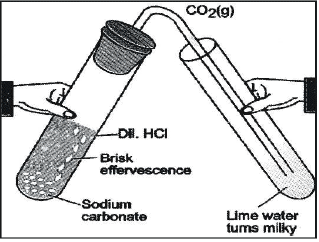(i) In a tube take small amount of solid sodium carbonate (Na2CO3). To this add a few drops of hydrochloric acid.
(ii) A colourless and odourless gas will evolve which shows that a chemical reaction has taken place. The gas will turn lime water milky when passed through it.

Q.5. Identify the type of reaction in the following examples:
(i) Na2SO4(aq) + Bacl2(aq) → BaSO4(s) + 2NaCl(aq)
(ii) Fe(s) + CuSO4(aq) → FeSO4(aq) + Cu(s)
(iii) 2H2(g) + O2(g) 2H2O(l)
Ans.

(i) It is an example of a double displacement reaction.
(ii) It is an example of a displacement reaction.
(iii) It is an example of combination reaction.

Q.6. Solid calcium oxide was taken in a container and water was added slowly to it.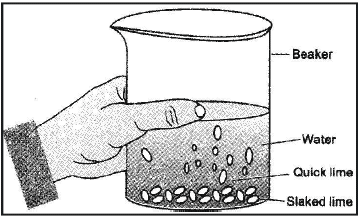(i) State two observations made in the experiment.
(ii) Write the name of the chemical formula of the product.

Ans.
(i) Water will start boiling and hissing noise will be produced.
(ii) Calcium hydroxide (slaked lime) will be formed.
CaO(s) + H2O(l) → Ca(OH)2(s) + Heat

Q.7. A housewife wanted her house to be whitewashed. She bought 10 kg of quick lime from the market and dissolved in 30 litres of water. On adding lime to water, she noticed that the water started boiling even when it was not being heated. Give the reason for her observation. Write the corresponding equation and name the product formed.
Ans.
A suspension of slaked lime also called calcium hydroxide is formed when water is added to quick lime.
CaO(s) + H2O(l) → Ca(OH)2(s) + Heat
Since the reaction is highly exothermic, the solution started boiling although it was not being heated. The suspension of slaked lime is allowed to cool for some time, preferably overnight. It is then decanted and the liquid obtained is used for whitewashing.

SOLVED QUESTIONS

Q.1. What is the basis of a balanced chemical equation?

OR

State the law on which a balanced chemical equation is based.

OR

State the law of conservation of mass.

OR

On what basis is a chemical equation balanced?
Ans. The basis of balanced chemical equation is the law of conservation of mass. Mass can neither be created nor destroyed in a chemical reaction in ordinary chemical and physical changes.
Example: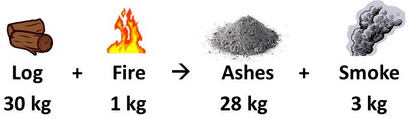Q.2. Would you call digestion of food in our body a chemical change?
Ans.
Yes. Digestion of food is an example of chemical change. Digestion is considered as chemical change because enzymes in the stomach and intestines break down large macro-molecules into simpler molecules so that the body can absorb the food easily.

Q.3. Balance the following chemical equation:
FeSO4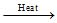Fe2O3 + SO2 + SO3
Ans.
Balanced chemical equation is
2FeSO4Fe2O3 + SO2 + SO3

Q.4. Balance the following chemical equation:
MnO2 + HCl → MnCl2 + Cl2 + H2O
Ans.
Balanced chemical equation is
MnO2 + 4HCl → MnCl2 + Cl2 + 2H2O

Q.5. Write a combination reaction in which two gases combine.
Ans.
Hydrogen and chlorine gases combine to form hydrogen chloride.
H2(g) + Cl2(g) → 2HCl(g)

Q.6. What change in colour is observed when white silver chloride is left exposed to sunlight? What type of chemical reaction is this?
Ans. When silver chloride is exposed to sunlight, the white colour of silver chloride changes to grey colour. This is a photochemical decomposition reaction.
2AgCl(s)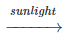2Ag(s) + Cl2(g)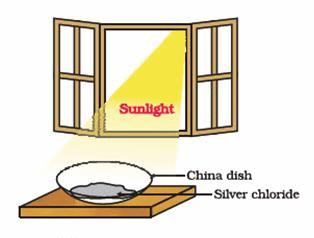Fig. Silver chloride under sunlight

Q.7. Why do we apply paint on iron articles?
Ans.
We apply paint on iron articles to protect them from corrosion.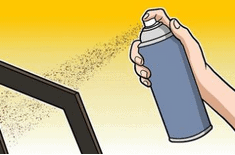Q.8. How can you help your mother in keeping the fried items so that they do not develop a bad smell and their shelf life is increased?
Ans. Since we cannot pack the fried objects in the atmosphere of nitrogen at home but we can increase their shelf life by keeping them in airtight containers. In this way they do not come in contact with oxygen. Their shelf life can further be increased by keeping them in fridge at low temperature.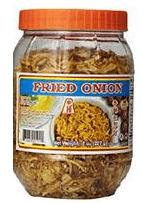Fig. Fried onion in airtight container

Q.9. A solution of a substance 'X' is used for whitewashing.
(i) Name the substance 'X' and write its formula.
(ii) Write the reaction of the substance 'X' named in (i) above with water.
Ans.
(i) Calcium oxide or quicklime, its formula is CaO.
(ii) CaO(s) + H2O(l) → Ca(OH)2(s)

Q.10. Write any two limitations of a chemical equation.
Ans. A chemical equation does not provide the following information:
(i) Whether the reaction is fast, slow or instantaneous i.e. the rate of the reaction is not shown by a chemical equation.
(ii) Whether the reaction goes to completion or is stopped in between i.e., the extent to which a reaction takes place is not known from a chemical equation.

Q.11. Balance the following equations:
(i) FeCl3 + NH4OH → Fe(OH)3 + NH4Cl
(ii) Na2S2O3 + I2 → Na2S4O6 + 2NaI
Ans.
Balanced equations:
(i) FeCl3 + 3NH4OH → Fe(OH)3 + 3NH4Cl
(ii) 2Na2S2O3 + I2 → Na2S4O6 + 2NaI

Q.12. Distinguish between an exothermic and an endothermic reaction. Amongst the following reactions, identify the exothermic reaction and the endothermic reaction:
(i) Heating coal in the air to form carbon dioxide.
(ii) Heating limestone in a lime kiln to form quicklime.
Ans. Exothermic reactions are those reactions in which heat is evolved.
Endothermic reactions are those that involve absorption of heat.
(i) Heating coal in air to form carbon dioxide is an exothermic reaction.
(ii) Heating limestone in a lime kiln to form quick lime is an endothermic reaction.

Q.13. What is an oxidation reaction? Give an example of an oxidation reaction. Is oxidation an exothermic or endothermic reaction?
Ans.
Reactions involving addition of oxygen are classified as oxidation reactions. For example, Magnesium reacts with oxygen to form magnesium oxide.
2Mg + O2 → 2MgO
Oxidation reactions are generally exothermic in nature.

Q.14. What is a redox reaction? When a magnesium ribbon burns in air with a dazzling flame and forms white ash, is magnesium oxidised or reduced? Why?
Ans.
Those reactions in which oxidation and reduction reactions occur simultaneously, are called redox reactions. In these reactions, one substance is oxidised and another substance gets reduced. When a magnesium ribbon burns to form white ash, magnesium metal is oxidised in this reaction because it combines with oxygen to form magnesium oxide.
2Mg(s) + O2 (g) 2MgO(s)

Q.15. In the reaction:
MnO2(s) + 4HCl(aq) → MnCl2(aq) + Cl2 + 2H2O(l)
Identify the oxidising and reducing agents.
Ans.
MnO2 loses oxygen, therefore it is reduced, thus it acts as an oxidizing agent.
HCl loses hydrogen. It itself gets oxidized, thus it acts as a reducing agent.
In the above reaction:
MnO2 is oxidizing agent.
HCl is a reducing agent.

Q.16. Give an example of a decomposition reaction. Describe an activity to illustrate such a reaction by heating.
Ans. Calcium carbonate decomposes on heating and forms calcium oxide and carbon dioxide.
CaCO3CaO + CO2

Q.17. Define a combination reaction. Give one example of a combination reaction which is also exothermic.
Ans.
Reactions in which two or more substances combine to form a new compound are called combination reactions. An example of this type of reaction is the combustion of magnesium in air, where magnesium combines with oxygen to form magnesium oxide, it is an exothermic reaction also.
2Mg(s) + O2(g) → 2MgO(s) + Heat

Q.18. Dilute solution of ammonium hydroxide is added to an aqueous solution of ferrous sulphate. Ferrous hydroxide is formed. What is the type of this reaction? Write chemical equations.
Ans. It is a double displacement reaction.
FeSO4(aq) + 2NH4OH(aq) → (NH4)2SO4(aq) + Fe(OH)2(s)

Q.19.
(i) What is observed when a solution of potassium iodide is added to a solution of lead nitrate taken in a test tube?
(ii) What type of reaction is this?
(iii) Write a balanced chemical equation to represent the above reaction.
Ans.
(i) A yellow precipitate of lead iodide will be formed.
(ii) This is a double displacement reaction.
(iii) Pb(NO3)2(aq) + 2KI(aq) → PbI2(s) + 2KNO3(aq)

Ques 20. Why does the blue colour of copper sulphate solution changes to green colour when an iron nail is dipped? Write chemical equations.
Ans. The blue colour of the copper sulphate solution changes to green colour because copper of copper sulphate is replaced by iron and forms ferrous sulphate which is green coloured.
Fe(s) + CuSO4(aq) → Cu(s) + Fe(SO4)(aq)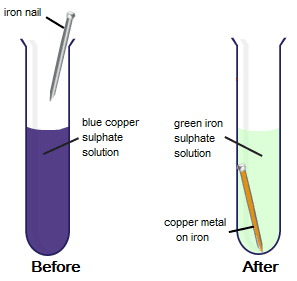Fig. iron nail when dipped into copper sulphate solution

Offer running on EduRev: Apply code STAYHOME200 to get INR 200 off on our premium plan EduRev Infinity!

,

,

,

,

,

,

,

,

,

,

,

,

,

,

,

,

,

,

,

,

,

,

,

,

,

,

,

;